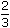# Aptitude - Alligation or Mixture

### Exercise :: Alligation or Mixture - General Questions

6.

A dishonest milkman professes to sell his milk at cost price but he mixes it with water and thereby gains 25%. The percentage of water in the mixture is:

 A. 4% B. 6% C. 20% D. 25%

Explanation:

Let C.P. of 1 litre milk be Re. 1

Then, S.P. of 1 litre of mixture = Re. 1, Gain = 25%.

 C.P. of 1 litre mixture = Re.100 x 1= 4 125 5

By the rule of alligation, we have:

C.P. of 1 litre of milk C.P. of 1 litre of water
Re. 1 Mean Price
 Re. 4 5
0
 4 5
 1 5Ratio of milk to water = 4 : 1 = 4 : 1. 5 5

 Hence, percentage of water in the mixture =1 x 100% = 20%. 5

7.

How many kilogram of sugar costing Rs. 9 per kg must be mixed with 27 kg of sugar costing Rs. 7 per kg so that there may be a gain of 10% by selling the mixture at Rs. 9.24 per kg?

 A. 36 kg B. 42 kg C. 54 kg D. 63 kg

Explanation:

S.P. of 1 kg of mixture = Rs. 9.24, Gain 10%.C.P. of 1 kg of mixture = Rs.100 x 9.24= Rs. 8.40 110

By the rule of allilation, we have:

 C.P. of 1 kg sugar of 1st kind Cost of 1 kg sugar of 2nd kind Rs. 9 Mean Price Rs. 8.40 Rs. 7 1.40 0.60Ratio of quantities of 1st and 2nd kind = 14 : 6 = 7 : 3.

Let x kg of sugar of 1st be mixed with 27 kg of 2nd kind.

Then, 7 : 3 = x : 27x =7 x 27= 63 kg. 3

8.

A container contains 40 litres of milk. From this container 4 litres of milk was taken out and replaced by water. This process was repeated further two times. How much milk is now contained by the container?

 A. 26.34 litres B. 27.36 litres C. 28 litres D. 29.16 litres

Explanation:

 Amount of milk left after 3 operations =401 - 43litres 40

 =40 x 9 x 9 x 9= 29.16 litres. 10 10 10

9.

A jar full of whisky contains 40% alcohol. A part of this whisky is replaced by another containing 19% alcohol and now the percentage of alcohol was found to be 26%. The quantity of whisky replaced is:

A.
 1 3
B.
 2 3
C.
 2 5
D.
 3 5

Explanation:

By the rule of alligation, we have:

 Strength of first jar Strength of 2nd jar 40% MeanStrength 26% 19% 7 14

So, ratio of 1st and 2nd quantities = 7 : 14 = 1 : 2Required quantity replaced = 2 3

10.

In what ratio must water be mixed with milk to gain 16% on selling the mixture at cost price?

 A. 1 : 6 B. 6 : 1 C. 2 : 3 D. 4 : 3

Explanation:

Let C.P. of 1 litre milk be Re. 1.

 S.P. of 1 litre of mixture = Re.1, Gain = 50 %. 3C.P. of 1 litre of mixture =100 x 3 x 1= 6 350 7

By the rule of alligation, we have:

C.P. of 1 litre of water C.P. of 1 litre of milk
0 Mean Price
 Re. 6 7
Re. 1
 1 7
 6 7Ratio of water and milk = 1 : 6 = 1 : 6. 7 7